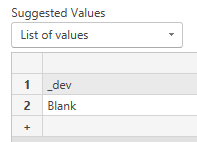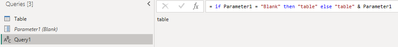cancel
Showing results for
Did you mean:Helper III

## insert empty value in parameter power query

Hello,

i would like to add a paramater in power query with list of value like _dev and empty value

when i select dev in my parameter i will have my table like this : table_dev

i when i select empty value i will have table

i tried with null, "", '', blank with no success 😞Do you know how to do it ?
Best regards

1 ACCEPTED SOLUTIONSolution Sage

I am assuming you want to return 'table1' if 'Blank' is selected with the parameter and 'table1_dev' if '_dev' is selected.
In that case you would need to change your code to

Table1 = dba{[Name= if parameter="blank" then "table1" else "table1" & parameter ,Kind="Table"]}[Data],....

5 REPLIES 5Helper III

I'm interested if you have any ideasSolution Sage

You cannot have a blank value in the parameter list.
To workaround you can add 'Blank' to the parameter list and then use an if statement to ignore the parameter if 'Blank' is selected.Helper III

hello @jgeddes

i tried this :

Table1 = dba{[Name="table1"& if parameter="blank" then "" else parameter ,Kind="Table"]}[Data],....

but id doesn't work 😕
thank you for you helpSolution Sage

I am assuming you want to return 'table1' if 'Blank' is selected with the parameter and 'table1_dev' if '_dev' is selected.
In that case you would need to change your code to

Table1 = dba{[Name= if parameter="blank" then "table1" else "table1" & parameter ,Kind="Table"]}[Data],....Helper III

Thank you so much @jgeddes